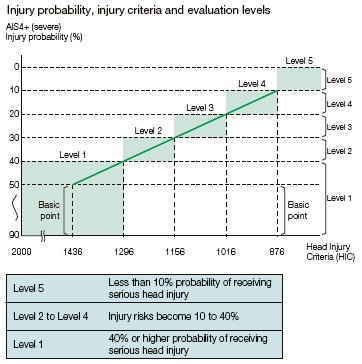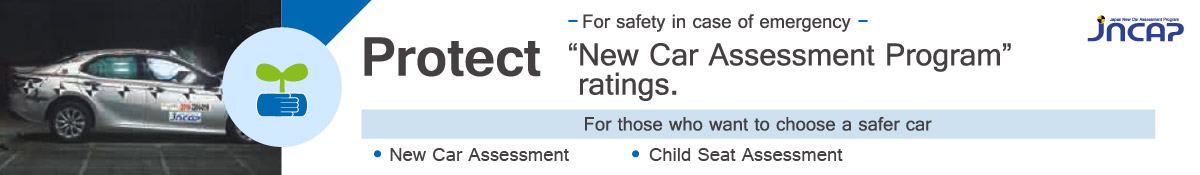# Pedestrian Head Protection Performance Tests

## Testing Methods

Car accidents in which pedestrians are hit by cars moving at a certain speed and their heads collided against car bonnets or front windshield are assumed.An adult or a child pedestrian’s head simulated impactor (head impactor) are projected toward the car bonnet, etc., from the testing machine. The impact received by the head impactors is measured and then evaluated using head injury criterion (HIC).
Discharge velocity is 40 km/h (equivalent to a vehicle colliding with a pedestrian at a speed of 50 km/h).
Before FY2015, it was 35 km/h (equivalent to a vehicle colliding with a pedestrian at a speed of 44 km/h).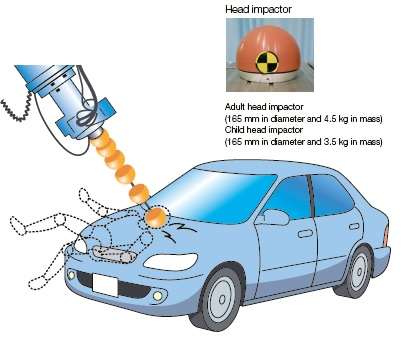### (1) Impact test area

The distance between the ground and the evaluated areas of the cars,i. e., Wrap Around Distance (WAD) is measured according to the length of the area where the pedestrians’ heads hit in accidents. Impact location area for adults and children’s head is set based on the data of actual accidents. In the crosswise direction, the side line of the impact test area is from the line obtained by tracing the contact points between a straight edge and the side of the bonnet where the straight edge contacts the bonnet bumper at 45 degrees (Bonnet Side Reference Line) to the inside half diameter of the head impactor.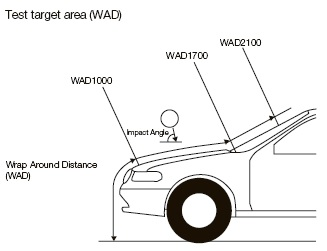### (2) Impact condition

Impact angle in the test is 65° for adult pedestrian’s head impactor and 50° for child pedestrian’s head impactor.
Before 2015, test vehicles were divided by the vehicle type and tests were conductedin each testing area under each impact condition.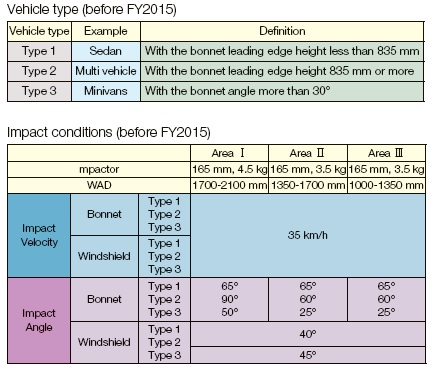## Evaluation Method

The impact position of the test is the point of intersection of two lines on the vehicle center line: one is drawn with 100 mm pitch from the point of WAD 1000 in front‐back direction and the other is the lines drawn with 100 mm pitch from the point of WAD 1000 in left-right direction. The injury criteria determined through testing are used and scored on an evaluation function (sliding scale). The total score of above tests are evaluated.

Impact area → HIC → Evaluation function → Score

Evaluation procedure before FY2015 was as follows.
Areas I, II, and III are divided into six sections from left to right (up until FY2010, Area III was divided into three sections). Each of the six sections is then subdivided into four quadrants.
In each of the 18 sections, one or two points that are thought to have the highest injury criteria are selected (the points must be in different quadrants). The injury criteria determined through testing are used as representative criteria and scored on an evaluation function (sliding scale).
The test scores are averaged to produce an average score for each section, and then the total average score is calculated.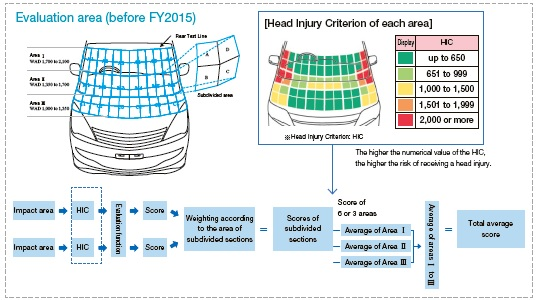## Interpretation of evaluation results

Evaluation is divided into five levels in the following manner. First, the total average scores are converted into the Head Injury Criteria (HIC). Then, assuming AIS4+ (severe head injury probability) of around 40% (HIC: 1295) as the basic point (= 1.54), the range for which the probability is approximately 10% (HIC: 876) or lower (3.14 points or higher) is categorized as Level 5, while the range between the point where AIS4+ is approximately 10% and that where AIS4+ is approximately 50% is divided into four equal parts, thus making five levels for evaluation.
For evaluation before FY2015, the basic point was 1.67 when AIS4+ (severe head injury probability) is approximately 50% (HIC: 1436) and the score when the probability is approximately 10% (HIC: 876) or lower was 3.33 points.
In regard to pedestrian protection, the pedestrian’s head injury criterion inevitably becomes higher than that of the passengers, given the current vehicle technology. As for the evaluation coverage, it is expanded to cover wider range where certain lifesaving effects are expected in order to promote development of pedestrian protection technology.